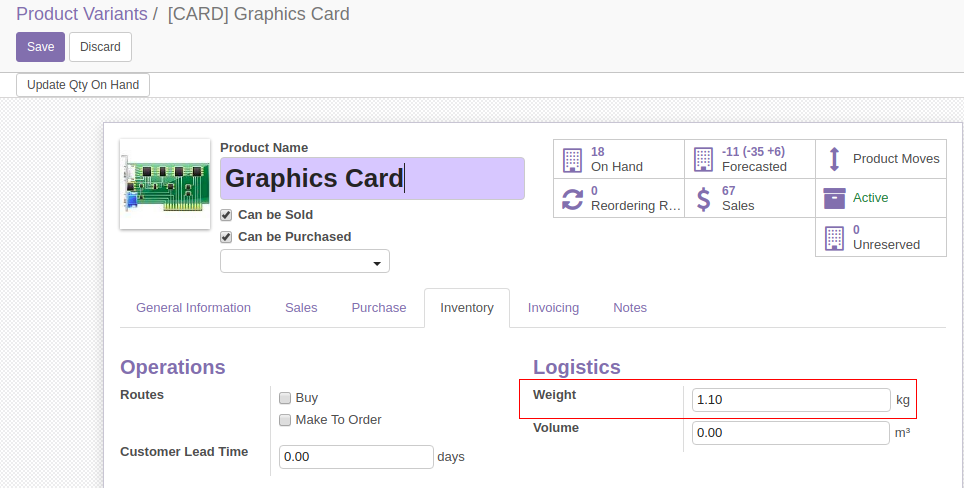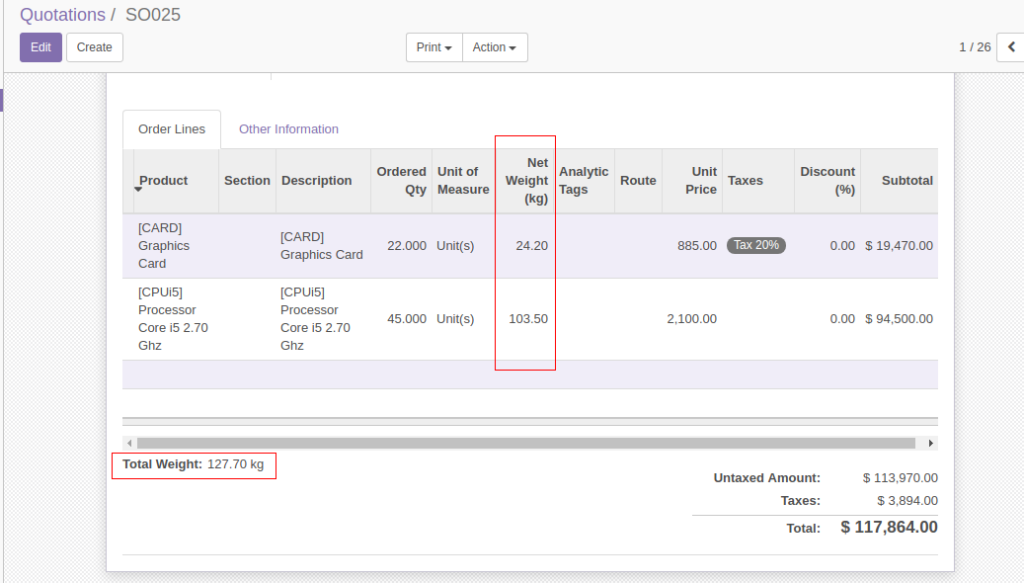• Get In Touch
India: +91 484 4050570, +91 90486 54482
Home » Apps » Sales Order Weight

# Sales Order Weight

This module from BroadTech IT Solutions manages to calculate total weight of a sale order, which is the sum of individual weights of each unit of the products in the order.

The product form has a field ‘Weight’, which stores the weight of the product in Kg.The module adds a field ‘Net Weight’ in sale order line, which calculates the Order line weight based on Order line Quantity and Unit of Measure. The new field ‘Total Weight’ in Sale Order would be the sum of sale order line weights.

The calculation uses the following formula:

Sale_order_line_net_weight = (line_product_weight * line_product_uom_qty / line_product_uom_factor)

Total_weight = sum(Sale_order_line_net_weight)